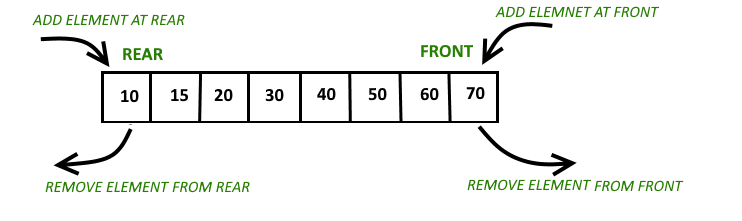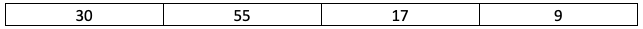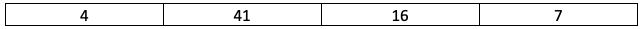×

Search anything:

# Use Deque in C++ STL

#### Software Engineering C++ standard template libraryGet this book -> Problems on Array: For Interviews and Competitive Programming

The deque in the STL of C++ is a dynamically resizing container to implement a double-ended queue data structure. Double ended queues are a special case of queues where insertion and deletion operations are possible at both the ends.### Definition in `<deque>`

std::deque is defined as follows under `<deque>` header file:

``````template <class T, class Allocator = std::allocator<T>> class deque;
``````

### Declaration

A deque with name a storing integers is declared as follows:

``````deque<int> a;
``````

### Functions

Assume deque a to be:and deque b to be:Some of the prominent functions under the `<deque>` header file are:

### Note

• All pop and push operations are ```O(1)``` operations.

### Illustration of Deque

``````#include <iostream>
#include <deque>

using namespace std;

void display(deque <int> x)
{
deque <int> :: iterator it;
for (it = x.begin(); it != x.end(); ++it)
cout << ' ' << *it;
cout << '\n';
}

int main()
{
deque<int> a;
a.push_back(10);
a.push_front(20);
a.push_back(30);
a.push_front(15);
cout << "The deque a is : ";
display(a);

cout << "\na.size() : " << a.size();
cout << "\na.front() : " << a.front();
cout << "\na.back() : " << a.back();

cout << "\na.pop_front() : ";
a.pop_front();
display(a);

cout << "\na.pop_back() : ";
a.pop_back();
display(a);

return 0;
}
``````
``````\\Console output
The deque a is :     15    20    10    30

a.size() : 4
a.front() : 15
a.back() : 30
a.pop_front() :     20    10    30

a.pop_back() :     20    10
``````

### Question

#### True or false: Deque is dynamically resizing

True
False
Memory is allocated based on size of deque.